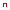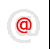Apache-Talk @lexa.ruInet-Admins @info.east.ruFilmscanners @halftone.co.uknginx-ru @sysoev.ruПИШИТЕПИСЬМААРХИВ :: Security-alerts

### [security-alerts] Fwd: Breaking RSA: Totient indirect factorization

```
Только мне кажется, что это бред, по причине того что

- Repeat "a = a^n mod m" with n from 2 to m, saving all the results in
a table until a == 1 (Statement 4).

означает выполнение гораздо большего числа операций, чем прямой перебор,
да еще и с хранением в памяти или не только мне?

--This is a forwarded message
From: gandlf <gandlf@xxxxxxxxx>
To: bugtraq@xxxxxxxxxxxxxxxxx <bugtraq@xxxxxxxxxxxxxxxxx>
Date: Thursday, November 15, 2007, 12:59:42 AM
Subject: Breaking RSA: Totient indirect factorization

===8<==============Original message text===============
Breaking RSA: Totient indirect factorization
=============================================

Author: Alex Bassas Serramia

(I'm very sorry for my poor english but it's not my first language).

Introduction
------------

This document tries to expose an algorithm that allows the RSA
modulus' totien factorization and then
breaking RSA.

RSA algorithm
-------------

RSA algorithm is generated the following way:

1) m = p*q            -> RSA modulus
2) t = (p-1)*(q-1)    -> totien(m)

3) (e*d) mod t = 1 mod t

4) a^e mod m = b
5) b^d mod m = a

e = public key
d = private key

RSA strength
------------

Equation (3) shows that is possible to recover private key "d" knowing
public key "e" and totient "t".

"a^n mod m" sequence
--------------------

To know about totien we have to examine the sequence "a^n mod m", one
sample is "2^n mod 11" (n from 1 to 11)
with totien 10:

2, 4, 8, 5, 10, 9, 7, 3, 6,*1, 2

At "n=10" we have one "1" because "a^totien(m) mod m" is always one
(Euler's theorem).

The sequence "3^n mod 11" has the same totien 10:

3, 9, 5, 4,*1, 3, 9, 5, 4,*1, 3

but we have two "1", "n=5" y "n=10" (totien), in this case we can
observe the cyclic nature of the "a^n mod m"
because we always have the same list of numbers before each "1".

"a^n mod m = 1" equation
------------------------

The cyclic nature of the "a^n mod m" sequence take us to the first statement:

1) - The exponent's values of the "a^n mod m = 1" solutions are always
totien's divisors.

The sequence "3^n mod 11" has "5" and "10" as solutions, they are
totien's divisors (totien(11) = 10).

3, 9, 5, 4,*1, 3, 9, 5, 4,*1, 3

Maximazing "a^n mod m = 1" solutions
------------------------------------

The second statement is:

2) - If "x" is a totien's divisor  then "a^x^n mod m = 1" will
multiply the
"a^n mod m = 1" solutions by "x".

Ex.: The "2^n mod 11" sequence has one "1"

2, 4, 8, 5, 10, 9, 7, 3, 6,*1, 2

"2^5^n=32^n", "32^n mod 11" produces 5 ones:

10,*1, 10,*1, 10,*1, 10,*1, 10,*1, 10

"a^n mod m = 1" limit
---------------------

The third statement is:

3) - If x is not yet a totien's divisor then "a^x^n mod m = 1"
will have the same
solutions that "a^n mod m = 1" but with the values permuted.

Ex.: The "2^n mod 11" sequence has one "1"

2, 4, 8, 5, 10, 9, 7, 3, 6,*1, 2

"2^2^n= 4^n", "4^n mod 11" has two "1"

4, 5, 9, 3,*1, 4, 5, 9, 3,*1, 4

"4^2^n= 16^n", "16^n mod 11" is still having two "1" but with the
values permuted.

5, 3, 4, 9,*1, 5, 3, 4, 9,*1, 5

Ending the sequence
--------------------

The last statement is:

4) - If "a" contains by power all the totien's divisors then
"a^n mod m" will
always be "1".

Ex.: "2^2^5^n= 1024^n mod 11

*1,*1,*1,*1,*1,*1,*1,*1,*1,*1,*1

Euler's extension
-----------------

This statement is a consequence of the statement number 3 but I don't
use it in the algorithm.

5) - If "n" is greater than the biggest number of the
coincidents totient's
divisors then:

a^((n-1)(t*(t+1)/2)) mod m = 1 mod m    (t = totien(m))

Algorithm
---------

- Repeat "a = a^n mod m" with n from 2 to m, saving all the results in
a table until a == 1 (Statement 4).
- Examine the table from end to begining printing "n" if the number of
"ones" is divided by "n" (Statements 1,2,3),

Impact
------

PKI vendors must change modulus generator algorithms to discard totients with
lower factors. Current certificates can be factorized in lower time
than expected
and compromised, vendors must review each one separately.

Credits
--------

Alex Bassas serramia
Barcelon (SPAIN)

Sample
------

/*

(c) Alex Bassas Serramia,
Barcelona,
SPAIN.

*/

#ifdef WIN32
#include <windows.h>
#include <io.h>
#else
typedef long long ULONG64;
#define TRUE  (-1)
#define FALSE (0)
#endif
#include <stdio.h>
#include <time.h>

ULONG64 getrand (void) {
ULONG64 n,num;

for (n=0;n<8;n++) {
num = (num << 8) | (rand()%256);
}
return (num);
}

ULONG64 expmod (ULONG64 x,ULONG64 n,ULONG64 m) {
ULONG64 r = 1;

while (n) {
if (n&1) {
r = (r*x)%m;
n = n - 1;
}
x = (x*x)%m;
n = n / 2;
}
return (r);
}

int isprime (ULONG64 p) {
ULONG64 k,a;

for (k=0;k<8;k++) {
a = getrand() % p;
if (expmod(a,p-1,p) != 1) {
return (FALSE);
}
}
return (TRUE);
}

ULONG64 value (ULONG64 bits) {
ULONG64 n;

n = 1 << bits;
return (n);
}

ULONG64 getprime (ULONG64 bits,ULONG64 mbits) {
ULONG64 num,m;

m = value(mbits);
do {
num = getrand();
if (bits < 64) {
num %= value(bits);
}
}
while ((num<m) || (num<=1) || !isprime(num));
return (num);
}

struct s_reg {
ULONG64 base;
ULONG64 exp;
ULONG64 res;
};

int num_reg (FILE *f) {
int l;

fseek (f,0,SEEK_END);
l = ftell(f);
return (l/sizeof(struct s_reg));
}

int go_reg (FILE *f,int num) {
fseek(f,num*sizeof(struct s_reg),SEEK_SET);
return (TRUE);
}

int read_reg (FILE *f,int num,struct s_reg *r) {
if (go_reg(f,num)) {
}
return (TRUE);
}

int write_reg (FILE *f,int num,struct s_reg *r) {
if (go_reg(f,num)) {
fwrite (r,sizeof(struct s_reg),1,f);
}
return (TRUE);
}

void delete_table (char *name) {
FILE *f;

f = fopen (name,"wb");
if (f != NULL) {
fclose(f);
}
}

void expand_table (char *name,ULONG64 m) {
FILE *f;
int l;
struct s_reg r;

f = fopen (name,"a+b");
if (f != NULL) {
l = num_reg (f);
if (!l) {
r.base = 2;
r.exp = 2;
r.res = expmod (r.base,r.exp,m);
write_reg (f,0,&r);
l = 1;
}
while (r.res != 1) {
r.base = r.res;
r.exp++;
r.res = expmod (r.base,r.exp,m);
write_reg (f,l++,&r);
}
fclose (f);
}
}

void reverse_table (char *name,ULONG64 m) {
FILE *f;
struct s_reg a,b;
int l,n;
ULONG64 r,e;

f = fopen (name,"rb");
if (f != NULL) {
l = num_reg (f);
e = 1;
for (n=l-1;n>=0;n--) {
r = expmod (a.base,e,m);
if (r != 1) {
printf ("reverse\texp = %I64i\r\n",a.exp);
e *= a.exp;
}
}
fclose (f);
}
}

#define TABLE   "test.dat"
#define P_BITS  32

int main (int argc,char *argv[],char *envp[]) {
ULONG64 p,q,m,t;

srand((unsigned)time(NULL));
p = getprime(P_BITS/2,0);
printf ("p = %I64i\r\n",p);
q = getprime(P_BITS/2,0);
printf ("q = %I64i\r\n",q);
m = p*q;
printf ("m = %I64i\r\n",m);
t = (p-1)*(q-1);
printf ("t = %I64i\r\n",t);
delete_table (TABLE);
expand_table (TABLE,m);
reverse_table (TABLE,m);
return (0);
}
===8<===========End of original message text===========

--
~/ZARAZA
Электрические шоки очень полезны для формирования характера. (Лем)

```Copyright © Lexa Software, 1996-2009.